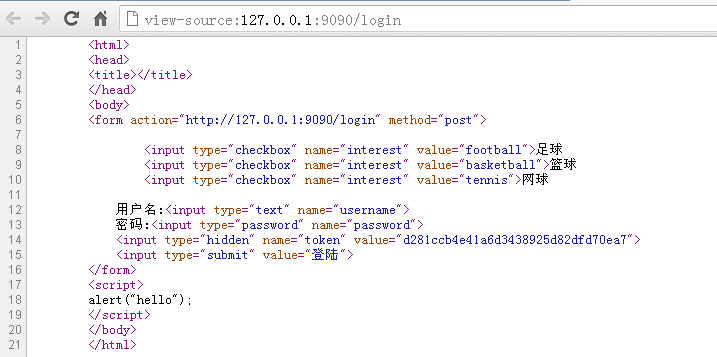# 4.4 防止多次递交表单

``````
<input type="checkbox" name="interest" value="football">足球
<input type="checkbox" name="interest" value="tennis">网球

<input type="hidden" name="token" value="{{.}}">
<input type="submit" value="登陆">
``````

``````
func login(w http.ResponseWriter, r *http.Request) {
fmt.Println("method:", r.Method) //获取请求的方法
if r.Method == "GET" {
crutime := time.Now().Unix()
h := md5.New()
io.WriteString(h, strconv.FormatInt(crutime, 10))
token := fmt.Sprintf("%x", h.Sum(nil))

t.Execute(w, token)
} else {
//请求的是登陆数据，那么执行登陆的逻辑判断
r.ParseForm()
token := r.Form.Get("token")
if token != "" {
//验证token的合法性
} else {
//不存在token报错
}
}
}
``````